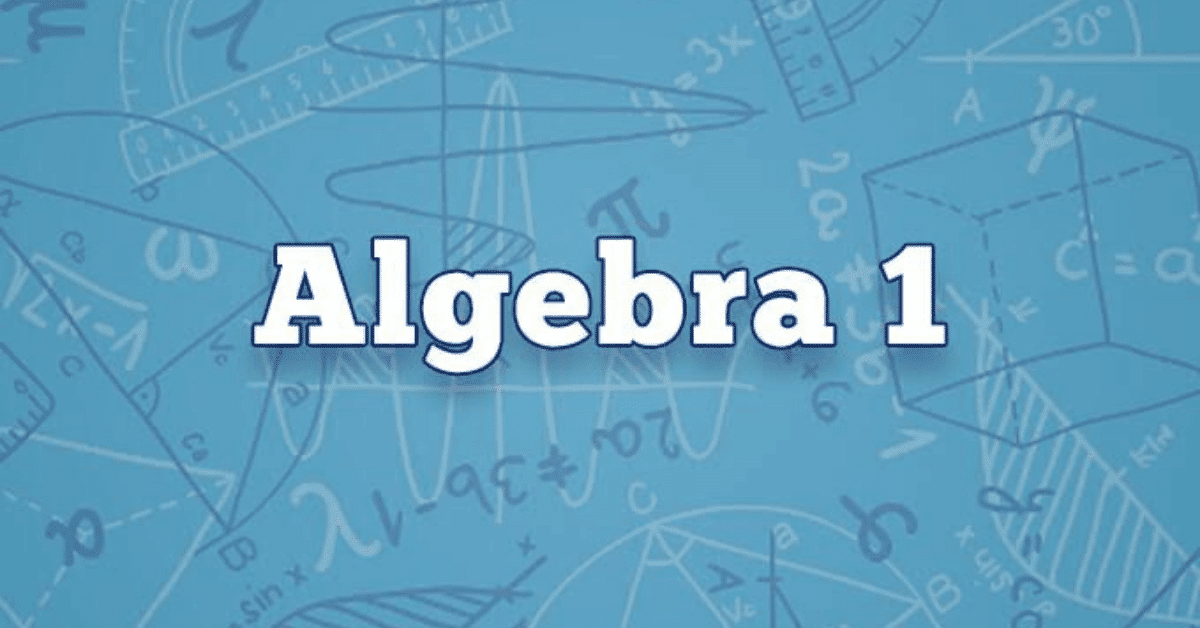Math 002

Algebra 1

Algebra 1 aims to improve math proficiency and problem solving skills to prepare students for higher mathematics such as Geometry and Algebra 2. Topics include:

• Algebraic foundations
• Variables and Expressions
• Ratios and percentages
• Functions and graphing
• Linear equations and inequalities